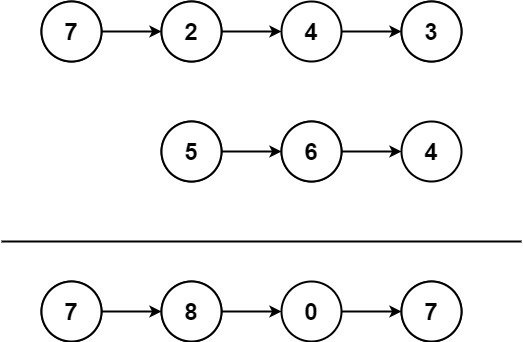# GeetCode Hub

You are given two non-empty linked lists representing two non-negative integers. The most significant digit comes first and each of their nodes contains a single digit. Add the two numbers and return the sum as a linked list.

You may assume the two numbers do not contain any leading zero, except the number 0 itself.

Example 1:```Input: l1 = [7,2,4,3], l2 = [5,6,4]
Output: [7,8,0,7]
```

Example 2:

```Input: l1 = [2,4,3], l2 = [5,6,4]
Output: [8,0,7]
```

Example 3:

```Input: l1 = , l2 = 
Output: 
```

Constraints:

• The number of nodes in each linked list is in the range `[1, 100]`.
• `0 <= Node.val <= 9`
• It is guaranteed that the list represents a number that does not have leading zeros.

Follow up: Could you solve it without reversing the input lists?

/** * Definition for singly-linked list. * public class ListNode { * int val; * ListNode next; * ListNode() {} * ListNode(int val) { this.val = val; } * ListNode(int val, ListNode next) { this.val = val; this.next = next; } * } */ class Solution { public ListNode addTwoNumbers(ListNode l1, ListNode l2) { } }